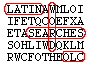## Word Search for Word Forms from OLC Chapter 29

You may wish to print out this exercise.

Read the following sentences and find/circle the Latin words corresponding to the underlined English words. Note that the Latin forms are inflected, so be sure to circle the complete form. Words may be horizontal, vertical, or diagonal.

 1. In which hand did she hold the rock? 2. Romulus founded Rome. 3. The dog walked out, carrying the bone. 4. They gave money to the learned gentlemen. 5. When did they arrive? 6. We convicted him on account of his crimes. 7. We won't do it unless we get extra credit. 8. No one is more welcome here than you. 9. Let's conduct our business in private. 10. I made a pie yesterday with the sweetest strawberries. 11. Always turn the other cheek. (cheek = gena, -ae, f.) 12. Superman flew over our house.

```z n c f h n e g o t i u m m v n
h d r d e g c a n i s u p r a t
z u p d e r d u l c i s r w i d
y l j c o w e l c o m e l d j x
u c h u a c l n c f t s i r r z
g i l l c n t z t l n d n v o n
w s l t a b e o a e n v e k w a
o s i r y a u m r o m e g q d s
s i b a g a r e c t p n o u o o
s m e u r l f u e o m l t a c s
i i n b a t e c r j j w i n t u
c s t n t e o p o r z x o d i p
u n e i i r s h d n g c r o s e
t m r s o a g x e j d m u r e r
d s a i r m a m c g d i m g c g
p k p r o p t e r q p b t f t j
```